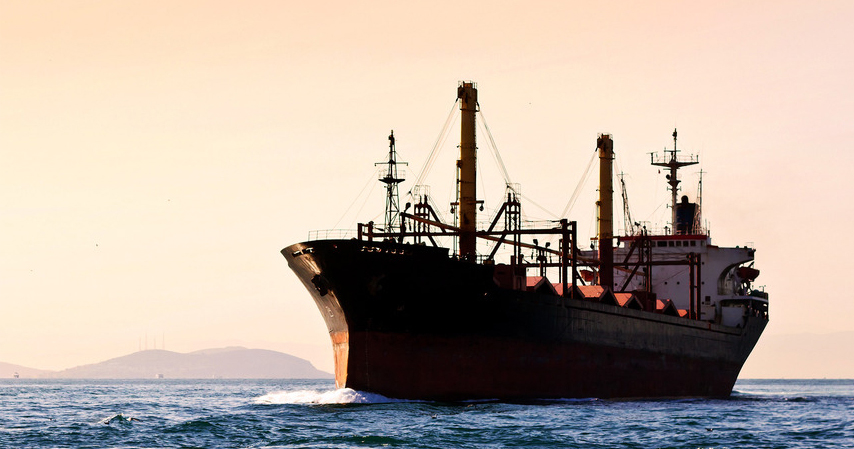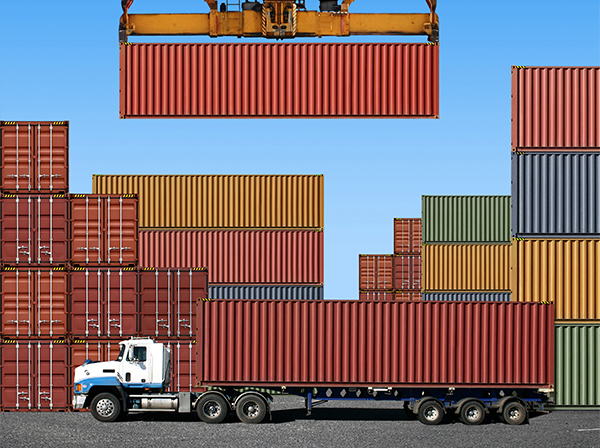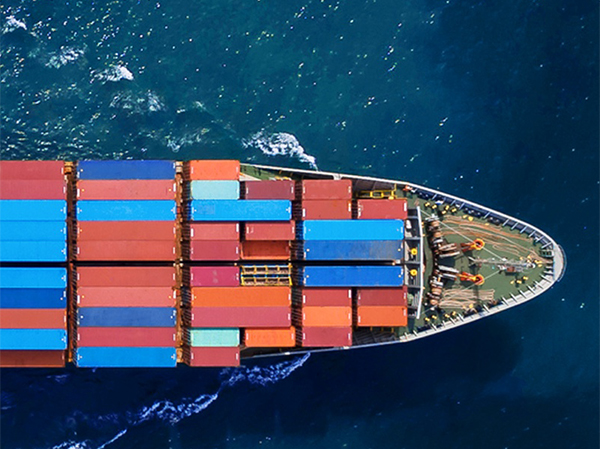Ocean Freight

CBM - Cubic Meter

CFT - Cubic Foot

THC - Terminal Handling Charges

LCL - Less than container load*

FCL - Full container load (20ft/40ft standard)

*LCL is based on cubic Meters.

Conversion:  L" * W" * H" / 1728 = Cubic ft , Cubic ft * 0.02832= Cubic m•Ground Freight

B-train- 2-20ft. trailers being pulled by one tractor

LTL- Less than a truckload

FTL - Full truckload

CWT- Per hundred pounds

PUP- 20ft. trailer

T/L - Trailer Load

Dimensional Freight- Subject to 10# per cubic ft.

• Calculation

Calculate Kilograms to Pounds

kgs x 2.2= pounds

Calculate Pounds to Kilograms

Pounds/2.2= kgs

Dimensional weight

L"xW"xH"/366= kgs
L"xW"xH"/166= pounds